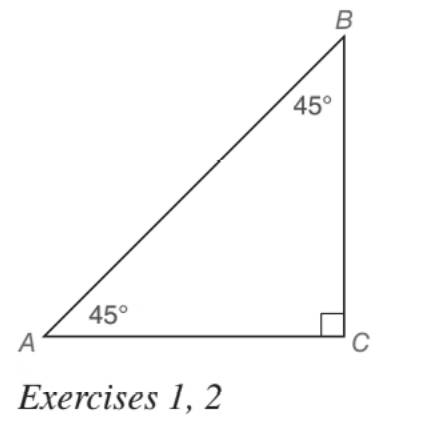Chapter 5.5, Problem 1EElementary Geometry For College St...

7th Edition
Alexander + 2 others
ISBN: 9781337614085

Solutions

Chapter
SectionElementary Geometry For College St...

7th Edition
Alexander + 2 others
ISBN: 9781337614085
Textbook Problem

For the 45 ° - 45 ° - 90 ° triangle shown, suppose that A C = a . Find: a) B C b) A BTo determine

a) To find:

BC, for the 45°-45°-90° triangle shown, suppose that AC=a.

Explanation

Approach:

For a right triangle for which the measure of the interior angles 45°, 45°, and 90°, if ‘a’ is the length of measure of one of the leg; opposite to the angle 45°, then the length of the other two sides is given by

Length of the other leg =a

Length of the hypotenuse =a2

In general

Length of the legs are equal.

Length of the hypotenuse =2× (Length of one of the legs)

Calculation:

Given,

A 45°-45°-90° triangle ABC with AC=a.

To determine

b) To find:

AB, for the 45°-45°-90° triangle shown, suppose that AC=a.

Still sussing out bartleby?

Check out a sample textbook solution.

See a sample solution

The Solution to Your Study Problems

Bartleby provides explanations to thousands of textbook problems written by our experts, many with advanced degrees!

Get Started

Find the mean, median, and mode for the following scores; 8, 7, 5, 7, 0, 10, 2, 4, 11, 7, 8, 7

Essentials of Statistics for The Behavioral Sciences (MindTap Course List)

If H() = sin , find H'() and H"( ).

Single Variable Calculus: Early Transcendentals

Proof Let x0 and n1 be real numbers. Prove that (1+x)n1+nx.

Calculus: Early Transcendental Functions (MindTap Course List)